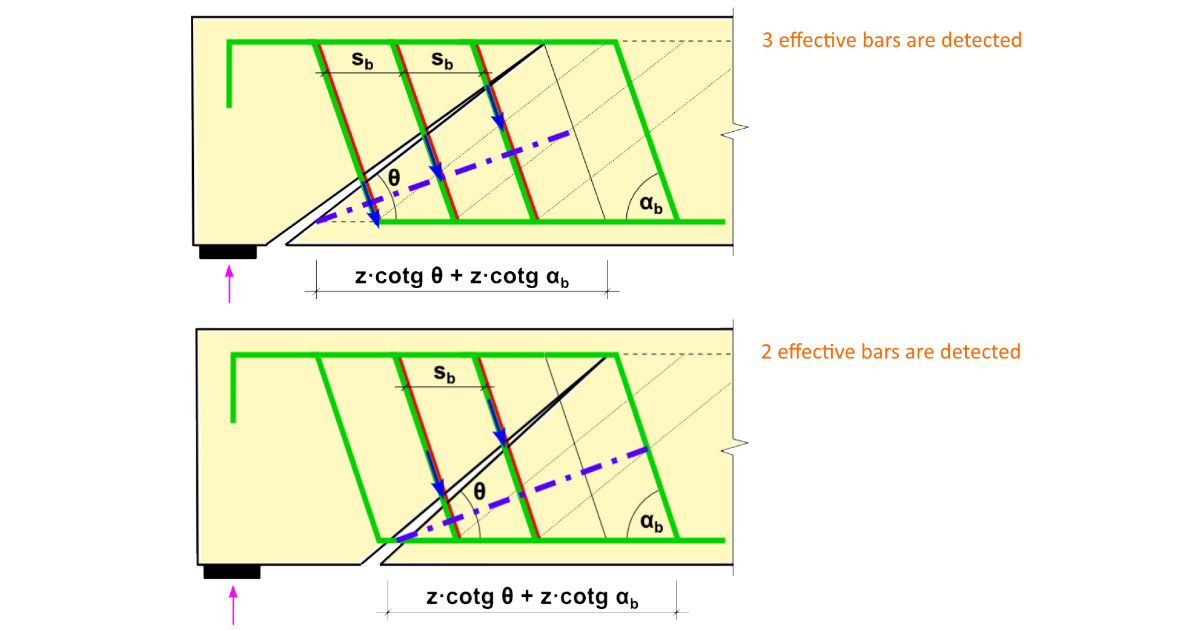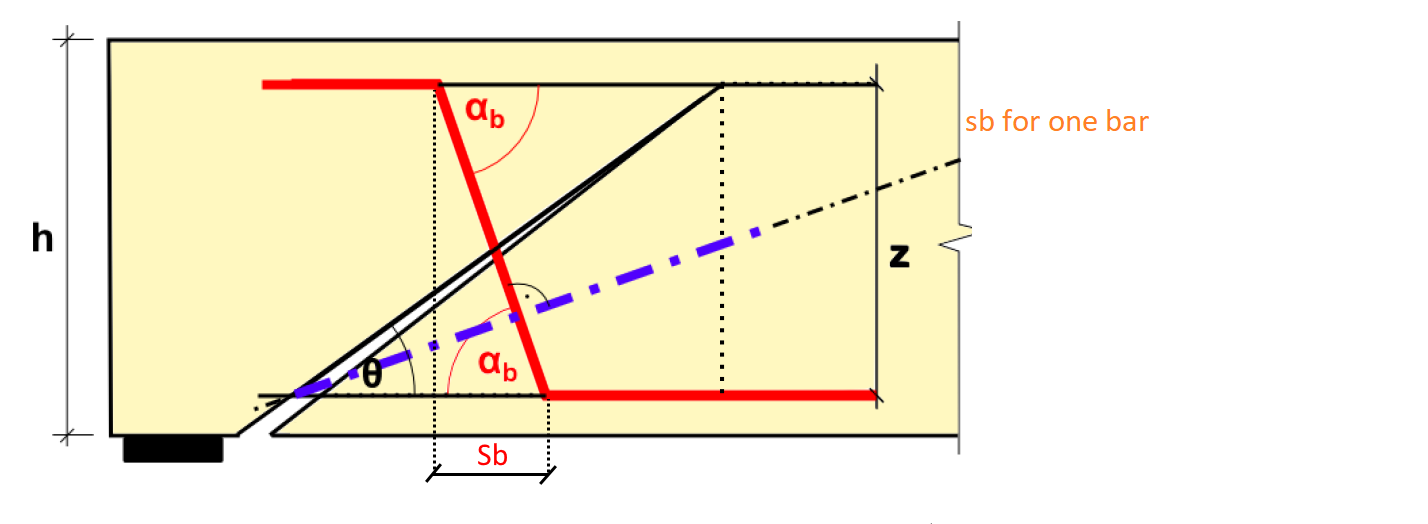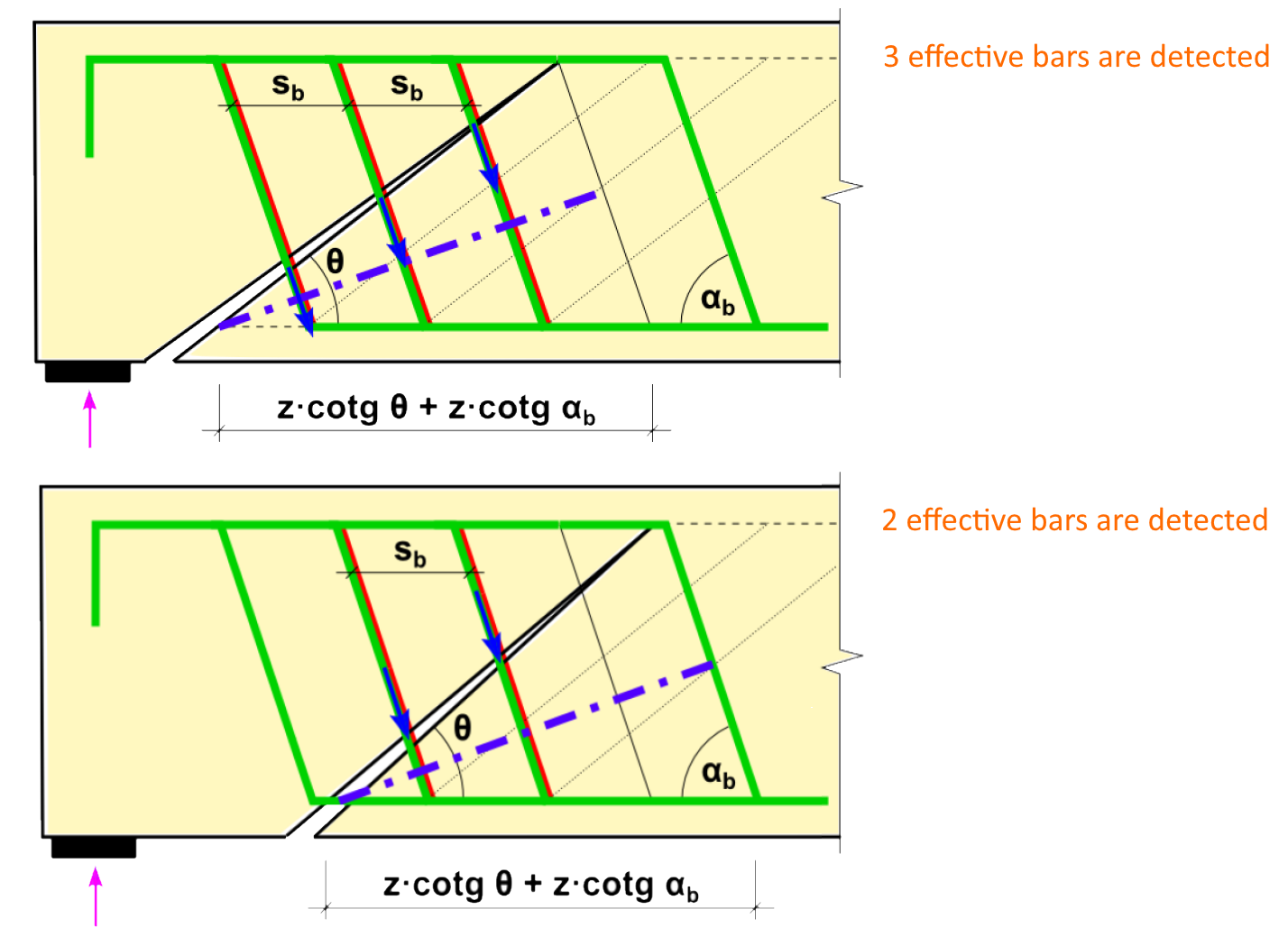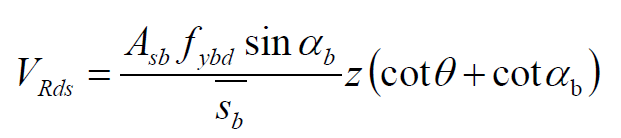### Choose language# How to correctly input bent-up bars?

$$The distance sb is defined as a projection of the inclined part to the direction of beam axis (or a rather effective length of bent up bar). For more layers of bent-up bars, the distance sb is defined as a horizontal distance between them. After this input, the automatic detection of effective bars in a projection of crack to the axis perpendicular to the geometry of bent-up bars is performed (blue dash-dotted line).The shear resistance of bent-up bars is defined by the following equation. If we use the unequal distance between the layers of bent-up bars, distance sb is averaged and used in the same equation.To consider smaller effectiveness of bent-up bars in areas of smaller crack width, there is an option to use the reduction by kb factor. The recommended value is 0,75 (generally <1).We should also check the maximal distance between bent-up bars according to the code.sb,max = 0,6 d (1 + cot α) (9.7N)$$